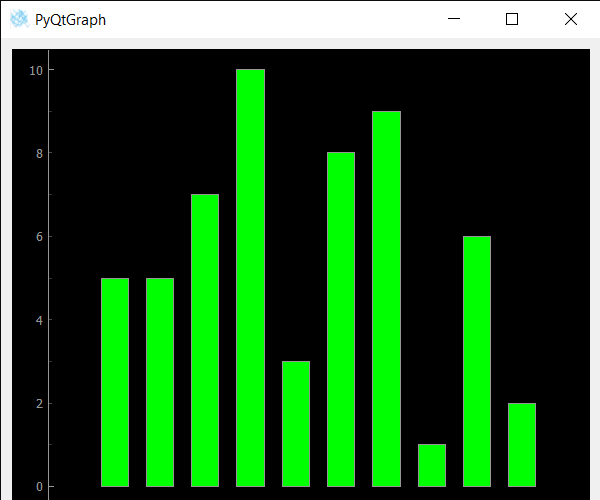Open In App

# PyQtGraph – Parent Changed Event for Bar Graph

In this article we will see how we can create a parent change event with the bar graph in the PyQtGraph module. PyQtGraph is a graphics and user interface library for Python that provides functionality commonly required in designing and science applications. Its primary goals are to provide fast, interactive graphics for displaying data (plots, video, etc.) and second is to provide tools to aid in rapid application development (for example, property trees such as used in Qt Designer).A bar chart or bar graph is a chart or graph that presents categorical data with rectangular bars with heights or lengths proportional to the values that they represent. The bars can be plotted vertically or horizontally. A vertical bar chart is sometimes called a column chart. This event get triggered when the parent of the bar graph is changed. Every time when bar graph is putted inside some other widget or frame it parent get changed, Main window is the top parent of the bar graph.
We can create a plot window and bar graph with the help of commands given below

```# creating a pyqtgraph plot window
window = pg.plot()

# creating a bar graph of green color
bargraph = pg.BarGraphItem(x=x, height=y1, width=0.6, brush='g')```

In order to do this we have modify the bar graph class, below is the class which can be used

```# Bar Graph class
class BarGraphItem(pg.BarGraphItem):

# constructor which inherit original
# BarGraphItem
def __init__(self, *args, **kwargs):
pg.BarGraphItem.__init__(self, *args, **kwargs)

# creating parent changed event
def parentChanged(self):

# print the message
print("Parent Changed")```

Below is the implementation

## Python3

 `# importing Qt widgets``from` `PyQt5.QtWidgets ``import` `*` `import` `sys` `# importing pyqtgraph as pg``import` `pyqtgraph as pg``from` `PyQt5.QtGui ``import` `*` `# Bar Graph class``class` `BarGraphItem(pg.BarGraphItem):` `    ``# constructor which inherit original``    ``# BarGraphItem``    ``def` `__init__(``self``, ``*``args, ``*``*``kwargs):``        ``pg.BarGraphItem.__init__(``self``, ``*``args, ``*``*``kwargs)` `    ``# creating parent changed event``    ``def` `parentChanged(``self``):` `        ``# print the message``        ``print``(``"Parent Changed"``)`    `class` `Window(QMainWindow):` `    ``def` `__init__(``self``):``        ``super``().__init__()` `        ``# setting title``        ``self``.setWindowTitle(``"PyQtGraph"``)` `        ``# setting geometry``        ``self``.setGeometry(``100``, ``100``, ``600``, ``500``)`  `        ``# icon``        ``icon ``=` `QIcon(``"skin.png"``)` `        ``# setting icon to the window``        ``self``.setWindowIcon(icon)` `        ``# calling method``        ``self``.UiComponents()` `        ``# showing all the widgets``        ``self``.show()` `    ``# method for components``    ``def` `UiComponents(``self``):` `        ``# creating a widget object``        ``widget ``=` `QWidget()` `        ``# creating a plot window``        ``plot ``=` `pg.plot()` `        ``# create list for y-axis``        ``y1 ``=` `[``5``, ``5``, ``7``, ``10``, ``3``, ``8``, ``9``, ``1``, ``6``, ``2``]` `        ``# create horizontal list i.e x-axis``        ``x ``=` `[``1``, ``2``, ``3``, ``4``, ``5``, ``6``, ``7``, ``8``, ``9``, ``10``]` `        ``# create pyqt5graph bar graph item``        ``# with width = 0.6``        ``# with bar colors = green``        ``bargraph ``=` `BarGraphItem(x ``=` `x, height ``=` `y1, width ``=` `0.6``, brush ``=``'g'``)` `        ``# bargraph.viewRangeChanged.connect(lambda: print("sss"))``        ``# add item to plot window``        ``# adding bargraph item to the plot window``        ``plot.addItem(bargraph)` `        ``# Creating a grid layout``        ``layout ``=` `QGridLayout()` `        ``# setting this layout to the widget``        ``widget.setLayout(layout)` `        ``# plot window goes on right side, spanning 3 rows``        ``layout.addWidget(plot, ``0``, ``1``, ``3``, ``1``)` `        ``# setting this widget as central widget of the main window``        ``self``.setCentralWidget(widget)`  `# create pyqt5 app``App ``=` `QApplication(sys.argv)` `# create the instance of our Window``window ``=` `Window()` `# start the app``sys.exit(App.``exec``())`

Output :```Parent Changed
Parent Changed```